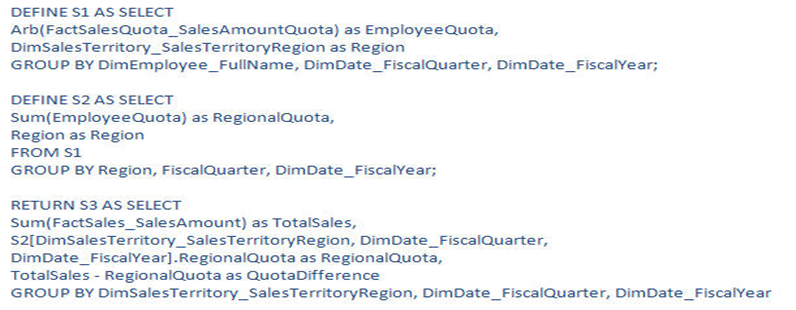# Endeca Server - Function

## Aggregate

• ARB. The ARB function returns an arbitrary value from a set of values. Generally to pick only one value
``````RETURN "Customers" AS
SELECT ARB�("CustomerAge") AS "Age"
GROUP BY "CustomerId"```
```
• AVG
• COUNT
• COUNTDISTINCT
• MAX
• MIN
• MEDIAN
• STDDEV
• SUM
• VARIANCE

## Scalar

Function Example
TO_DURATION (time function)
TRUNC Trunc(12345.67 ,-2) = 12300
POWER Power(2,3) = 8
LOG Log(10,1000) = 3
MOD Mod(10,3) = 1
ROUND Round(12.123,2) = 12.12
ABS ABS(-37) = 37
CEIL CEIL(45.37) = 46
COS COS(Pi/3) = .5
EXP EXP(1) = 2.7182818… (e)
FLOOR FLOOR(15.8) = 15
LN LN(2.7182818) = .999999 or LN(e) = 1
SIN SIN(Pi/6) = .5
SQRT SQRT(16) = 4
TAN TAN(Pi/4) = 1
SIGN SIGN(234.23) = 1 or SIGN(-123)=-1

## Date/Time

Require a data type of DateTime, Time, Duration

• DateTime - Integer representing the # of milliseconds since Jan 1, 1970
• Time –Integer representing the # of milliseconds since midnight
• Duration – Integer representing the # of elapsed milliseconds

Discover MoreEndeca Server - Endeca Query Language (EQL)

EQL is the Endeca Query Language that permits you to retrieve data. view manager Simple EQL statements can be broken down into five primary components: Clauses are not case-sensitive Standard and...